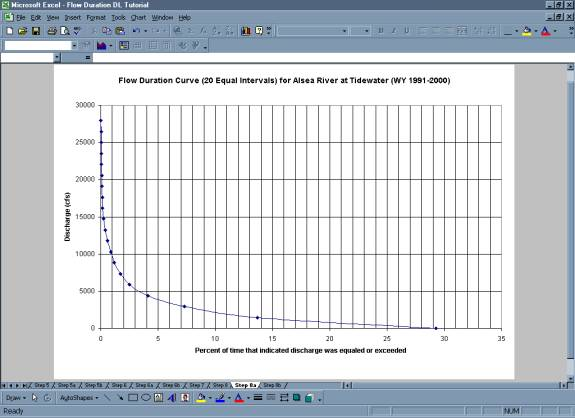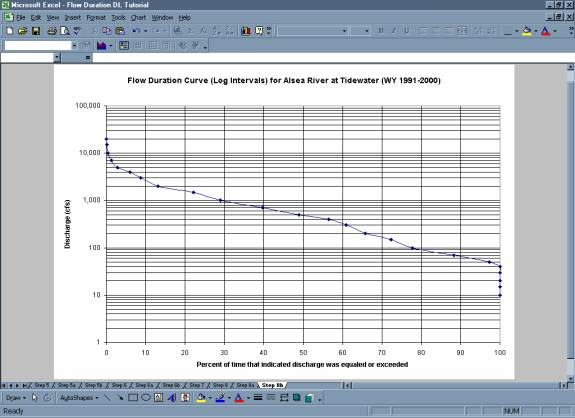# Analysis Techniques: Flow Duration Analysis Tutorial

## Step 1:  Select the time step value (day, month, etc.) and download the chronological record of discharge

• For the Alsea Example and Tutorial, the analysis will be done using a daily time step.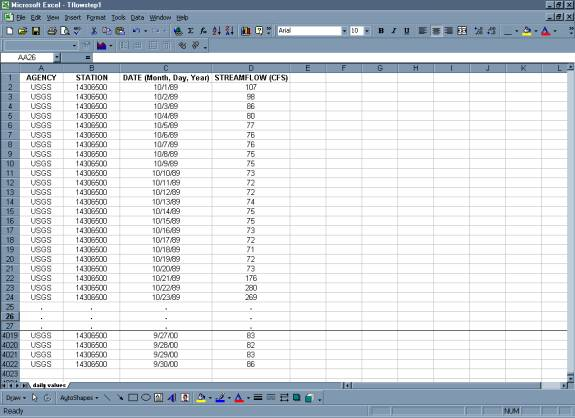## Step 2:  Compute the total number of time step intervals in the period of record.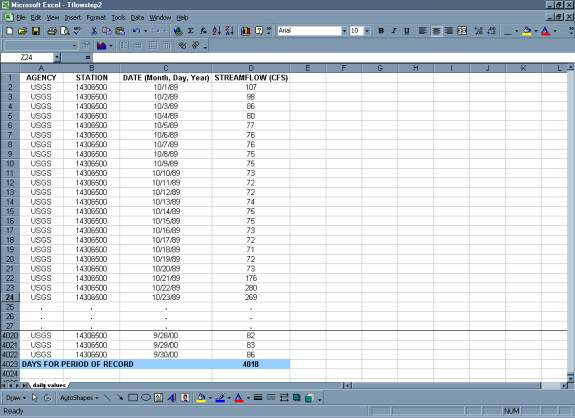## Step 3:  Rank discharge by magnitude.

• Use the "sort" command to rank the entries by discharge, from largest to smallest.

• Create a new column called "Rank". Use the excel "rank" function to determine the rank of each discharge in the period of record.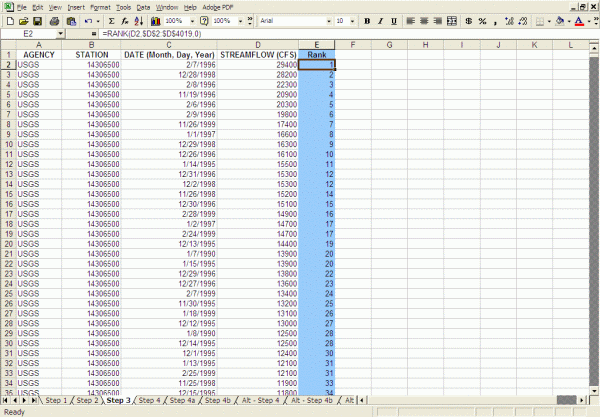## Step 4: Calculate the percent of time that each discharge is equaled or exceeded.

• Create a new column called "Exceedence Probability". As noted on the flow duration page, the exceedence probability can be calculated as follows:

P = 100 * [ M / (n + 1) ]

P = the probability that a given flow will be equaled or exceeded (% of time)
M = the ranked position on the listing (dimensionless)
n = the number of events for period of record (dimensionless)

Create a formula to calculate this value for each record using the information from Step 2 (=(E2/('Step 2'!\$D\$4020+1))*100).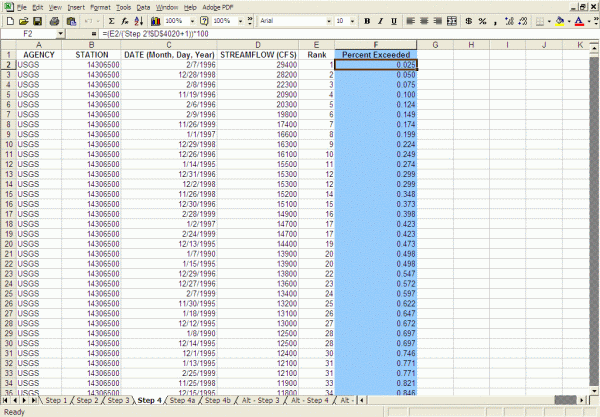• Graph the "exceedence probability" versus the discharge. The graph can have either linear or logarithmic axes.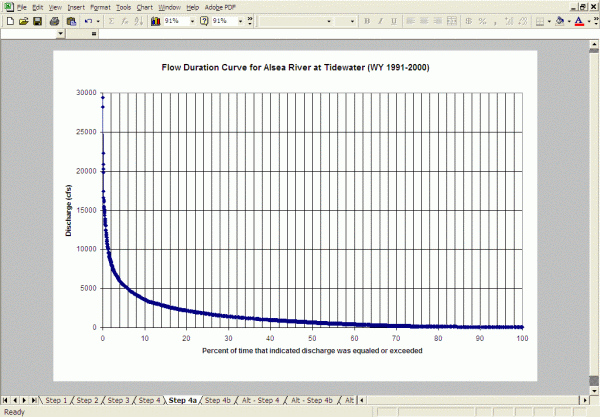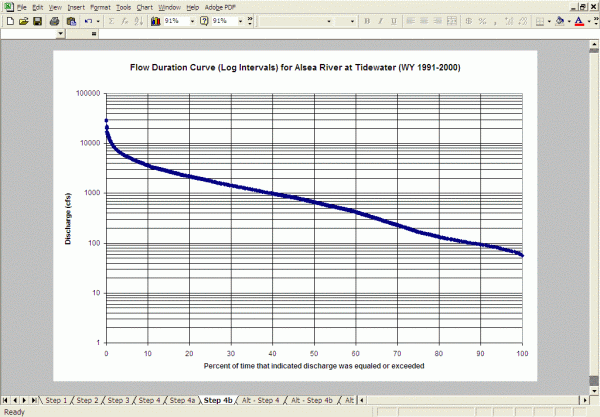## Alternative Approach:

Before spreadsheet programs were common, flow duration curves were constructed by grouping the data into discharge size classes (bins). This avoided the once time-consuming tasks of sorting, ranking, and graphing the individual discharge records. With modern spreadsheet programs, these steps can be done quickly and flow duration curves can be created as we described above.

However, some flow duration analyses are still made by grouping data into size classes. The steps below will guide you through this alternative approach.

## Alternative Step 4:  Divide the range of average values into classes.

• Calculate the average value of the variable of interest within each time step (average daily value) for the period of record and note the largest and smallest of these average values.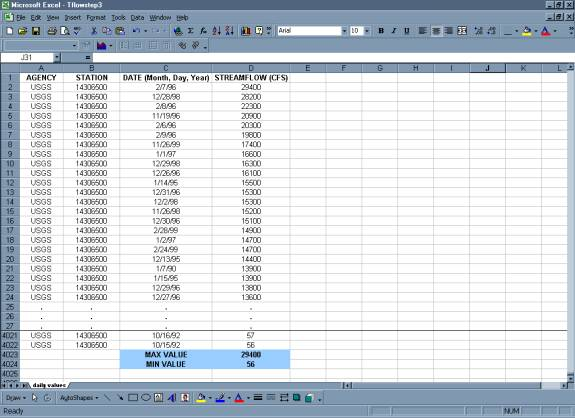• It is recommended to have between twenty to thirty class intervals for the period of record. Classes can either be equal interval or based on log cycles. Log cycles are often used to sort data because the probability of choosing appropriate interval spacing is higher than if the data were separated into 20 to 30 equal classes. If improper intervals are chosen, the amount of information the flow duration curve can provide is diminished.
• For the equal interval method, determine the discharge range for each class by dividing the max discharge value by the desired number of size classes. In the tutorial data, the max discharge value is 29400 cfs. That value divided by 20 is 1470. So for twenty size classes with equal intervals in each class, the smallest size class will be discharges between 0-1470 cfs. The second size class will be 1471-2940 cfs and so on, up to the max value.
• For classes based on log cycles, select classes of discharge values based on a spacing of 1, 1.5, 2, 3, 4, 5, 7, 10, or on multiples of 10 of these values. For the tutorial data, the size classes will be 10-14 cfs, 15-19 cfs, 20-29 cfs on up to 20,000-29,999 cfs.
• Use the ranked data to count the total number of occurrences of values in each class.

20 Equal Class Intervals: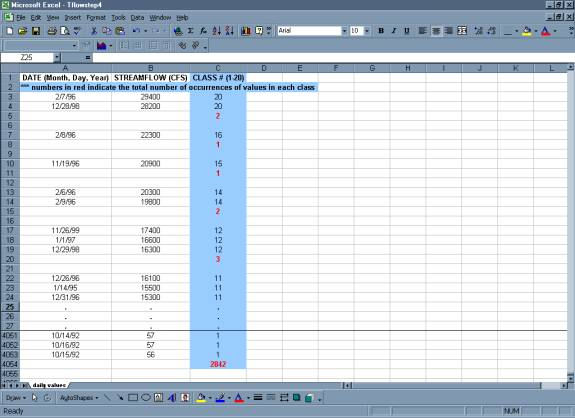Using Log Cycles: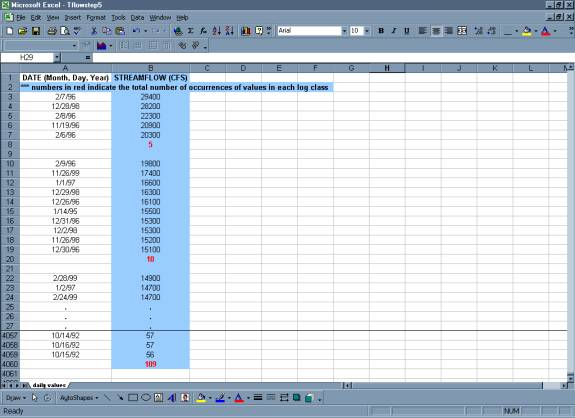## Alternative Step 5:  Summarize the results in a table.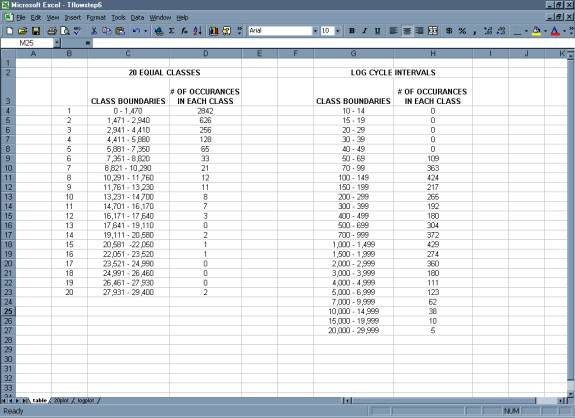• A plot of the total number of occurrences in each class versus discharge gives a frequency distribution.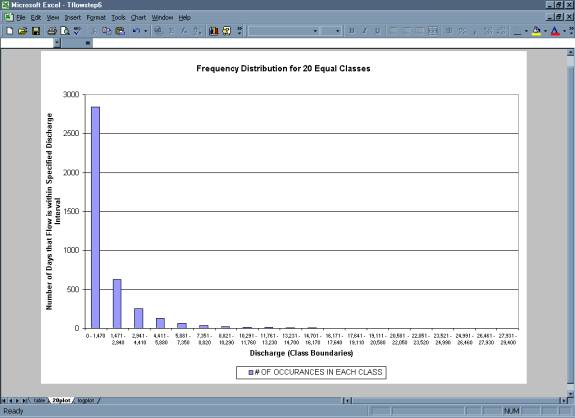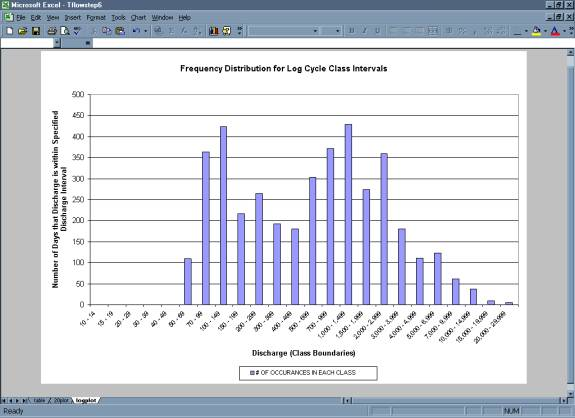## Alternative Step 6:  Beginning with the upper boundary of the highest class, add up the total number of values that are greater than the lower boundary for each successive class.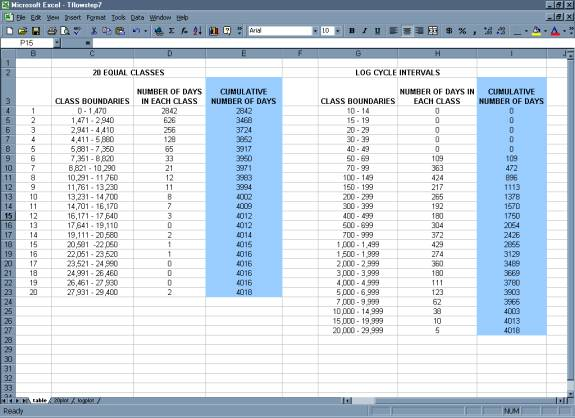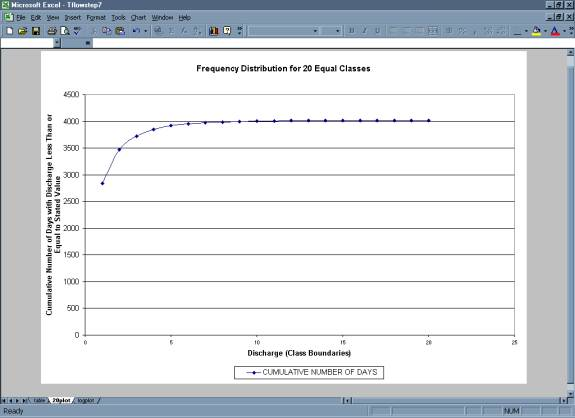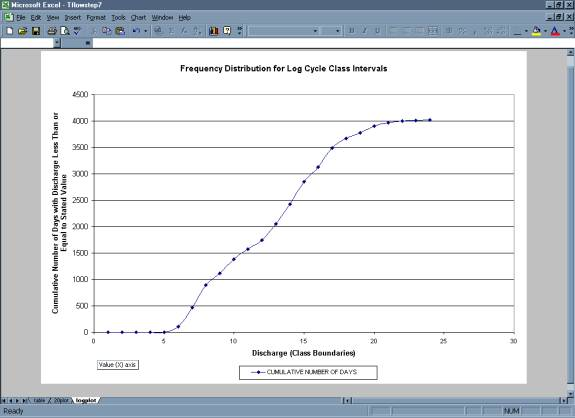## Alternative Step 7:  The cumulative number of occurrences is converted to a percentage of the time.

• Divide the values developed above by the total number of time steps from Step 2;  this gives the frequency with which the lower values of each class have been equaled or exceeded in the period of record.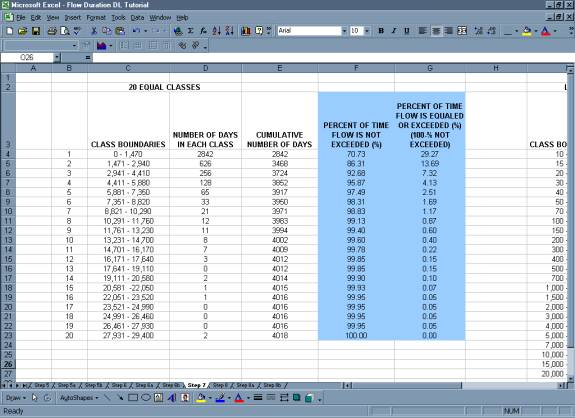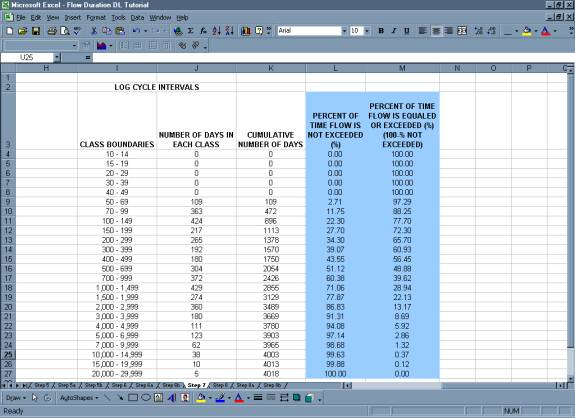## Alternative Step 8:  Finally the diagram is turned so that discharge is given on the vertical axis and exceedence frequency is given on the horizontal axis.